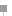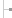Working with operators in Formula language for Lotus Notes

Here's a list of all the different operators in Formula language for Lotus Notes.

The following is tip #4 from "A Formula language for Lotus Notes introduction -- 7 tips in 7 minutes," excerpted from Chapter 5 of the book Lotus Notes Developer's Toolbox, published by IBM Publishing.

Working with operators

Operators are used to compare or equate values and for mathematic calculations. The following table lists the primary oprators used in formulas.

 Operator Operator usage or description - Used in subtraction or to represent a negative number ! Represents a logical NOT != Represents not equal & Represents a logical AND * Used in multiplication / Used in division : Used to separate a list of values := Used to assign a value to a variable | Represents a logical OR + Used in addition or to represent a positive number < Represents less than <= Represents less than or equal to <> Represents not equal = Represents equal =! Represents not equal > Represents greater than >< Represents not equal >= Represents greater than or equal toA Formula language for Lotus Notes introductionHome: IntroductionTip 1: What is Lotus Formula Language?Tip 2: Working with variables in Formula language for Lotus NotesTip 3: Lotus Notes Formula language keywordsTip 4: Working with operators in Formula language for Lotus NotesTip 5: Lotus Notes Formula language general syntax rulesTip 6: What are Lotus Notes Formula language functions and commands?Tip 7: Working with text strings in Formula language for Lotus NotesThis chapter excerpt from Lotus Notes Developer's Toolbox, by Mark Elliott, is printed with permission from IBM Publishing, Copyright 2006. Click here for the chapter download or purchase the book here.

Dig Deeper on Lotus Notes 5

• Favorite iSeries cheat sheets

Here you'll find a collection of valuable cheat sheets gathered from across the iSeries/Search400.com community. These cheat ...

Close